deutsch     english    français     Print

## 3.2 FOR LOOPS### INTRODUCTION

 You often have to count during repetition. For that, you need a variable in the repetition block that changes by a certain value in every iteration of the loop. It is easier to do this using a for structure than it is with a while structure. You must first understand the range() function. In the simplest case, range() has a single parameter (also called stop value) and provides a sequence of natural numbers that starts with 0 and ends with the last number before the stop value. You can try this out with a few examples. If, for example, you execute a program with the single statement print range(10), the numbers  [0, 1, 2, 3, 4, 5, 6, 7, 8, 9] are written in the output window. Try it out with a few different parameters. As you can see, in our example, the stop value 10 is not included in the list; it rather indicates how many list elements there are. PROGRAMMING CONCEPTS: Iteration, for structure, nesting of for loops

### FAMILY OF LINES

 You can draw a cool curve with 20 lines using this for structure. ```from gpanel import * makeGPanel(0, 20, 0, 20) for i in range(21): line(i, 0, 20, i) ``` Highlight program code (Ctrl+C copy, Ctrl+V paste)### MEMO

 The statement for i in range(n) runs through the numbers from 0 to n-1, so in other words, a total of n numbers. The places of consolidation of the lines form a quadratic Bézier curve.

### RANGE() WITH TWO PARAMETERS

 The range function can also have two parameters. In this case, the first parameter is the start value of the list and the second is the stop value, which is, however, not included in the list. If, for example, you write print range(2, 9), the numbers [2, 3, 4, 5, 6, 7, 8] are written in the output window. Try it out using a few other parameters. Using the following program, you draw lines in two colors with the start points on the x-axis from the coordinates -20 to 20. The endpoint of all lines is the point (0, 40).```from gpanel import *

makeGPanel(-20, 20, 0, 40)

for i in range(-20, 21):
if i < 0:
setColor("red")
else:
setColor("green")
line(i, 0, 0, 40)
```
Highlight program code (Ctrl+C copy, Ctrl+V paste)

### MEMO

 The loop for i in range(start, stop) with integer start and stop values begins at i = start and ends at i = stop - 1, where the loop counter i is increased by 1 each time it runs through the loop. Thereby you need to make start smaller than stop, otherwise the loop will never run.

### RANGE() WITH THREE PARAMETERS

 You can even call the range function with three parameters. In this case, the first parameter is the start value of the list, the second is the stop value, and the third is the change in value from one element to the next. This will help you to adjust the step size, which was previously always 1, to any situation. If, for example, you write print range(2, 15, 3), the numbers [2, 5, 8, 11, 14] are written in the output window. In the adjacent graphic you draw a pyramid standing on its peak with filled rectangles. The smallest rectangle has a width of 2, and the biggest has a width of 40.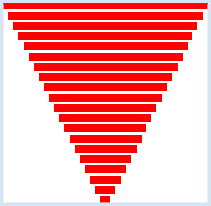```from gpanel import *

makeGPanel(0, 40, 0, 40)

setColor("red")
y = 1
for i in range(2, 41, 2):
move(20, y)
fillRectangle(i, 1.5)
y = y + 2 ```
Highlight program code (Ctrl+C copy, Ctrl+V paste)

### MEMO

 The loop for i in range(start, stop, step) begins with i = start and ends at a value that is less than stop. i is increased by step each time the program runs through the loop. You can also choose negative numbers for the values start, stop and step. I step is negative, i is reduced by step at every iteration; the last value is greater than stop.

### NESTED FOR LOOPS (Moiré)

 Closely drawn lines positioned above one another can produce an optical effect called the Moiré pattern. In a square, you draw lines from 10 points on the bottom edge to each of 10 points on the upper edge. Then you do the same from the left to the right edge.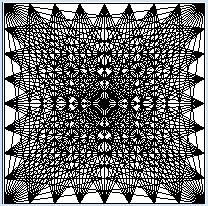```from gpanel import *

makeGPanel(0, 10, 0, 10)

for i in range(11):
for k in range(11):
line(i, 0, k, 10)
delay(50)
for i in range(11):
for k in range(11):
line(0, i, 10, k)
delay(50)
```
Highlight program code (Ctrl+C copy, Ctrl+V paste)

### MEMO

 The program might not be very easy to understand, but it is important. At best, you can assume that the loop variable i of the outer loop has a constant value (initially 0) . The inner loop runs with this value for the values k = 0 up to and including 10. Then i is set to 1 and the inner loops run again with this value i, etc. When using the command delay(millisec), the program waits for the given number of milliseconds so that you can observe how the pattern emerges gradually.

### EXERCISES

1.

You will get an even nicer graphic than the one seen in Example 1 if you use colors. Draw a second family of lines with a different color (Figure a).

 (a)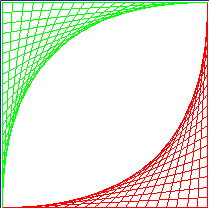(b)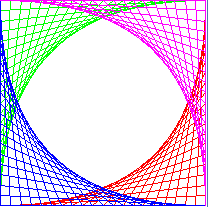The blue family of lines (Figure b) is drawn with line(i, 0, 0, 20 - i). Can you also program the purple one?

2.

Draw a family of circles.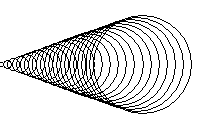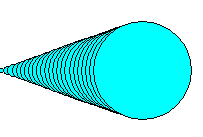You can draw the colored family of circles with the following procedure: First draw a filled circle with the radius y, then choose the color black and draw another circle with the same radius:

```   setColor("cyan")
fillCircle(y)
setColor("black")
circle(y)
```

3.
 In Example 3 we drew a pyramid standing upside down. Draw a "real" pyramid with three colors. In order to do so, you can use a for loop that counts down.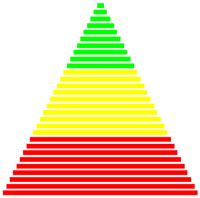.